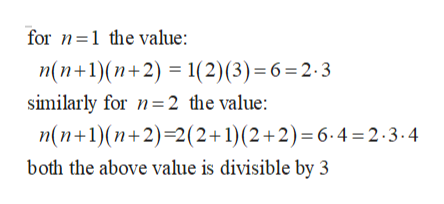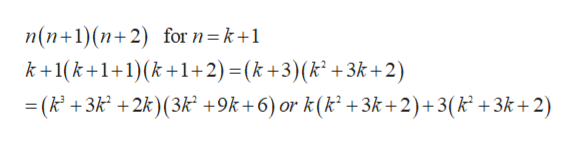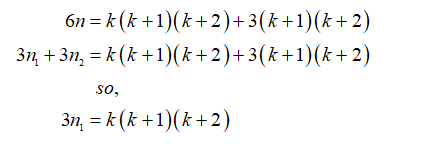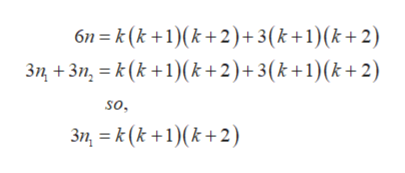Claim: For any natural number n, if 6 ∣ n, then 3 ∣ n.

Question

Claim: For any natural number n, if 6 ∣ n, then 3 ∣ n.

Step 1

Use the mathematical induction to show that the given statement For any natural number n, if 6  n, then 3  n” is true for all natural numbers n.

Let the number 6 can be written in the form n(n+1)(n+2) because n(n+1) (n+2) is always divisible by 6.

Using mathematical induction:help_outlineImage Transcriptionclosefor 1 the value: n(n+1)(n+2)1(2) (3) = 6 = 2 . 3 similarly for 2 the value: n(n1)(n+2)-2(2+1)(2+2) = 6.4=2.3-4 both the above value is divisible by 3 fullscreen
Step 2

let the statement is true for n = k so k(k+1)(k+2) is divisible by 3.

Then the statement n(n+1) (n+2) is always divisible by 6 is true for n = k+1.

So,help_outlineImage Transcriptionclosen(n+1) (n2) for n= k+1 k+1(k1+1k 1+2) =(k+3)(k +3k+2) = (k +3k +2k)(3k +9k+6) or k(k2 +3k+2)+3(k +3k+ 2) fullscreen
Step 3

So, the above statement is true as the above expression must be divisible by 6. And we have to show that the same expression is divisible by 3. The expression 3(k2+3k+2) is always divisible by 3 and the other expression k(k2+3k+2) = k(k+1)(k+2)

So,...help_outlineImage Transcriptionclose6n k(k +1)(k+2)+3(k +1)(k+ 2) 3+3n,(k1)(k + 2) + 3(k +1) (k+ 2) so. 3,k(k1)(k2) fullscreen

Want to see the full answer?

See Solution

Want to see this answer and more?

Our solutions are written by experts, many with advanced degrees, and available 24/7

See Solution
Tagged in

Math